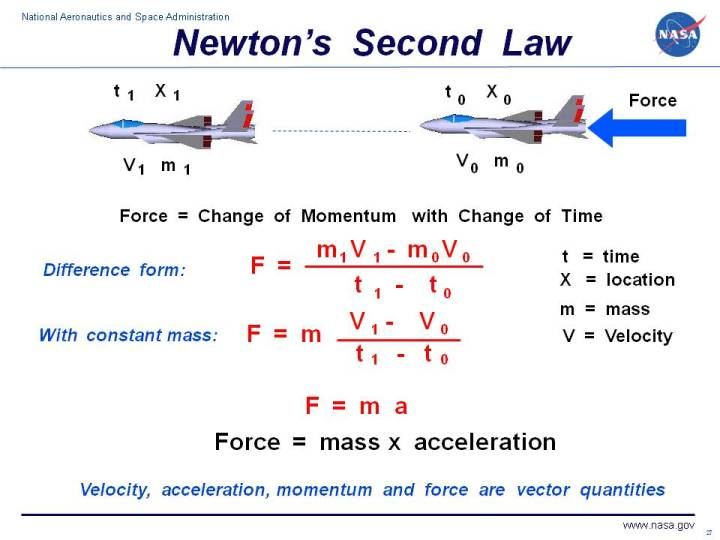+ Text Only Site
+ Non-Flash Version
+ Contact GlennThis page is intended for high school or middle school students. For younger students, a simpler explanation of the information on this page is available on the Kids Page. For college students there is another version.Sir Isaac Newton first presented his three laws of motion in the "Principia Mathematica Philosophiae Naturalis" in 1686. His second law defines a force to be equal to the change in momentum with a change in time. Momentum is defined to be the mass m of an object times its velocity V.

Let us assume that we have an airplane at a point "0" defined by its location X0 and time t0. The airplane has a mass m0 and travels at velocity V0. The airplane is subjected to an external force F and moves to a point "1", which is described by a new location X1 and time t1. The mass and velocity of the airplane change during the flight to values m1 and V1. Newton's second law can help us determine the new values of V1 and m1, if we know how big the force F is. Let us just take the difference between the conditions at point "1" and the conditions at point "0".

F = (m1 * V1 - m0 * V0) / (t1 - t0)

Newton's second law talks about changes in momentum (m * V) so, at this point, we can't separate out how much the mass changed and how much the velocity changed. We only know how much product (m * V) changed.

Let us assume that the mass stays a constant value equal to m. This assumption is pretty good for an airplane, the only change in mass would be for the fuel burned between point "1" and point "0". The weight of the fuel is probably small relative to the weight of the rest of the airplane, especially if we only look at small changes in time.. If we were discussing the flight of a baseball, then certainly the mass remains a constant. But if we were discussing the flight of a bottle rocket, then the mass does not remain a constant and we can only look at changes in momentum. For a constant mass m, Newton's second law looks like:

F = m * (V1 - V0) / (t1 - t0)

The change in velocity divided by the change in time is the definition of the acceleration a. The second law then reduces to the more familiar product of a mass and an acceleration:

F = m * a

Remember that this relation is only good for objects that have a constant mass. This equation tells us that an object subjected to an external force will accelerate and that the amount of the acceleration is proportional to the size of the force. The amount of acceleration is also inversely proportional to the mass of the object; for equal forces, a heavier object will experience less acceleration than a lighter object. Considering the momentum equation, a force causes a change in velocity; and likewise, a change in velocity generates a force. The equation works both ways.

The velocity, force, acceleration, and momentum have both a magnitude and a direction associated with them. Scientists and mathematicians call this a vector quantity. The equations shown here are actually vector equations and can be applied in each of the component directions. We have only looked at one direction, and, in general, an object moves in all three directions (up-down, left-right, forward-back).

The motion of an aircraft resulting from aerodynamic forces, aircraft weight, and thrust can be computed by using the second law of motion.

Activities:Guided Tours

Navigation ..Beginner's Guide Home Page+ Inspector General Hotline + Equal Employment Opportunity Data Posted Pursuant to the No Fear Act + Budgets, Strategic Plans and Accountability Reports + Freedom of Information Act + The President's Management Agenda + NASA Privacy Statement, Disclaimer, and Accessibility CertificationEditor: Nancy Hall NASA Official: Nancy Hall Last Updated: May 05 2015 + Contact Glenn